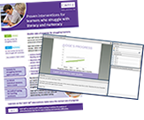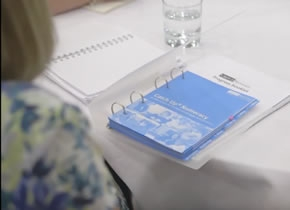### Find out moreRequest a full Catch Up® information brochure and details of our free information webinars

# News

### 8th February 2019 - Lynne’s logic – looking at 2019!Welcome back to a further instalment of Lynne’s logic. As I write this, we are into 2019 - an interesting number! 2019 is a multiple of 3; you will know this, because if you add the digits 2, 0, 1 and 9 it totals 12. Add these digits, 1 and 2, and the result is 3.

Any number whose digits total 3, 6 or 9 must be a multiple of 3 so, consequently, 2019 has a factor of 3. Therefore, it cannot be a prime number, as a prime number always has two factors: 1 and the number itself.

We know 2019 has factors of 1, 2019 and 3, and if we use 3 we can get the final factor 673, so 1, 3, 673 and 2019 are the four factors of 2019. Interestingly, 3 and 673 are both prime numbers, so 2019 is the product of two prime factors!

A challenge you could set your learners, would be to ask how many of the numbers from 0 to 10 can they make using 2, 0, 1 and 9 (though some of these are tricky!) To set you off, here is just one way of making 0:

0 x (2 + 1 + 9) = 0

So, that’s a very quick look at 2019 and what we can do with it! Next time, we will have an in-depth look at something that is often very difficult for our learners - Word Problems!